• 交换概论
• 交换网络

# 第一章 交换概论

## 通信网（三要素）

• 交换设备：包括各类交换机和交叉连接设备。
• 传输设备：包括用户线路、中继线路、信号转换设备。（如：双绞线、电缆、光缆、基站无线收发设备、光电转换器、卫星、微波等）
• 终端设备：包括电话机、智能终端、智能外设等用户终端设备。

## 交换方式的特点

### 电路交换

• 信息传送的最小单位是时隙：每个时隙采样 8bit，采样率 8kHz，每路通信速率 64kbps。
• 面向连接的工作方式（物理连接）：连接建立、信息传送、连接拆除。
• 同步时分复用（固定带宽分配）：时间划分为帧，帧划分为时隙。
• 无差错控制机制（实时性强）。
• 对通信信息不作处理（透明传输）。
• 流量控制基于呼叫损失制。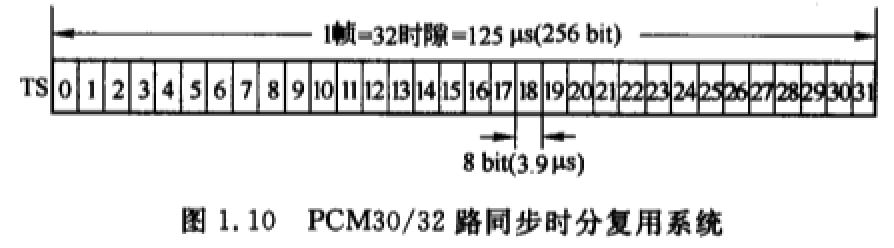### 分组交换

• 信息传送的最小单位分组：由分组头、用户信息构成，分组头含有选路和控制信息。
• 面向连接（虚电路）方式和无连接（数据报）方式。
• 统计时分复用，动态分配带宽。
• 有差错控制机制：CRC 校验、重发等（设于第二层和第三层协议中）。
• 信息传送不具有透明性：对通信信息作处理，如拆分、重组等。
• 流量控制基于呼叫延迟制。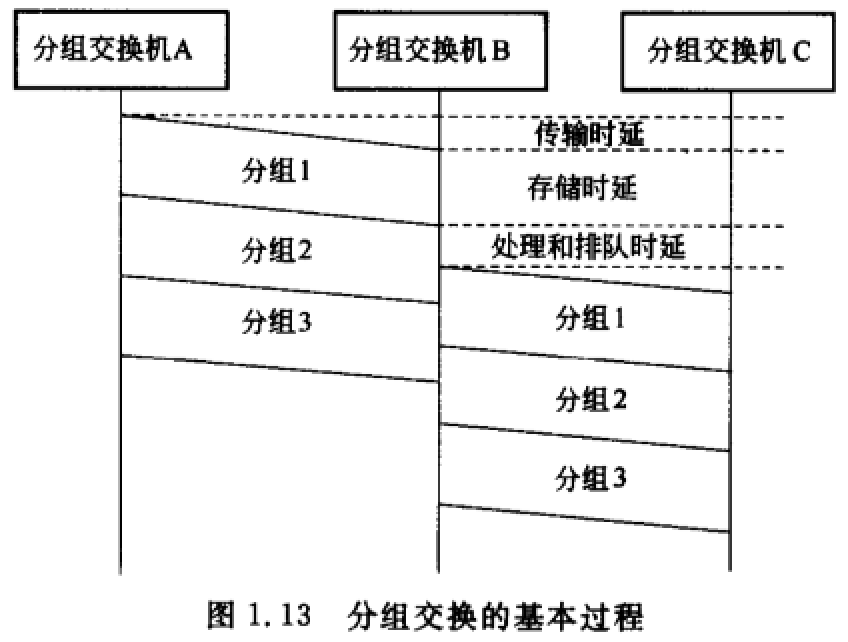ATM 交换 信元 固定
MPLS 交换 标记分组 可变

## 复用技术

### 同步时分复用

• 用于电路交换。
• 采用位置化信道：
• 根据数字信号在时间轴上的位置区别各路。无信息传送时，也会占用信道。
• 每帧定长。
• 信号经过复用器和分路器的时延（1~2 帧）。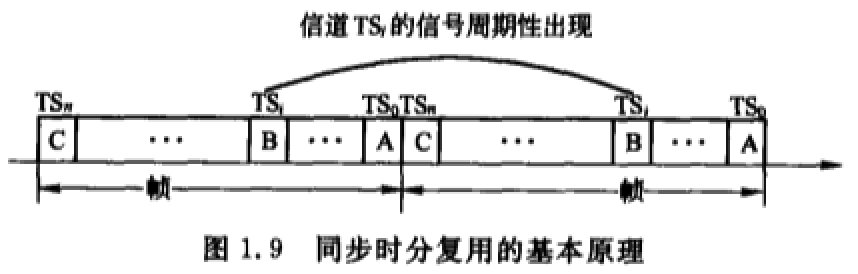### 统计时分复用

• 用于分组交换、ATM 交换。
• 采用标志化信道：使用分组头的标志区分各路通信。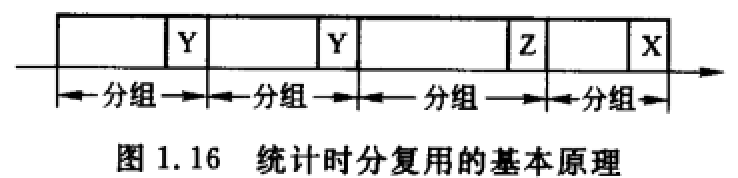#### 分组化时分复用

• 每个分组前附加标志码，标识分组的输出端或传送路径，与时间无关。
• 分组长度可变、分组头定界。
• 统计复用提高信道利用率。具有一定容量的排队存贮器，解决瞬间的出线冲突。
• 传输时延较小且稳定、处理时延与分组大小有关、排队时延与突发流量有关。

#### 异步时分复用

• 位置固定的标志化信道。
• 信元长度固定、插入空信元保持同步。
• 时延较为稳定。

## 连接类型

### 面向连接/无连接

• 无论物理或逻辑连接，通信分为三阶段：连接建立、传送信息、连接拆除
• 连接建立后，传送的所有信息均沿此路径传送，保证信息有序性。
• 信息传送的时延（比无连接）小。
• 对网络故障敏感。

• 没有连接建立过程，边选路边传送。
• 同一通信的信息到达目的地的路径无法预知，不保证信息有序性。
• 信息传送的时延（比面向连接）大。
• 对网络故障不敏感。

## 交换技术的种类和特点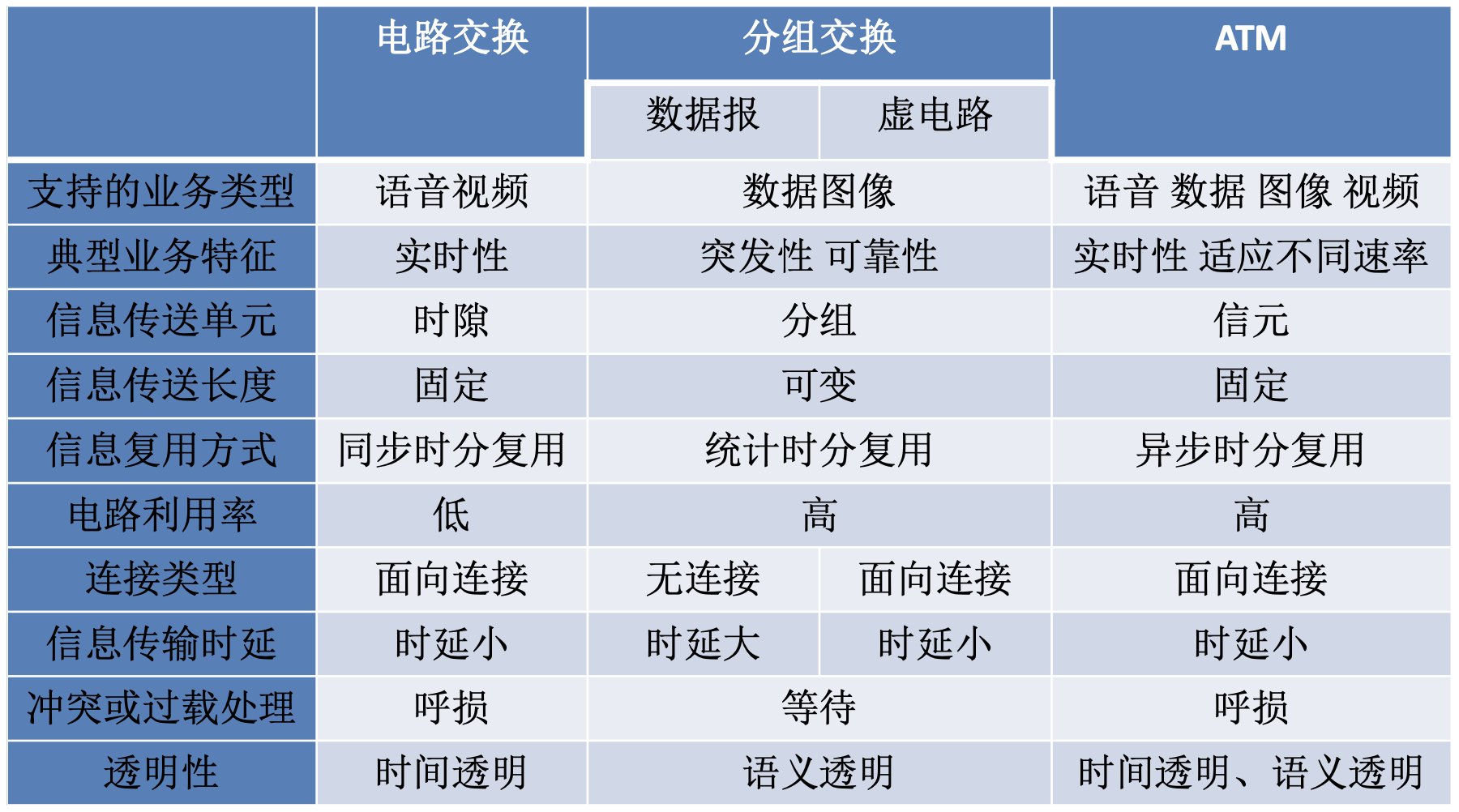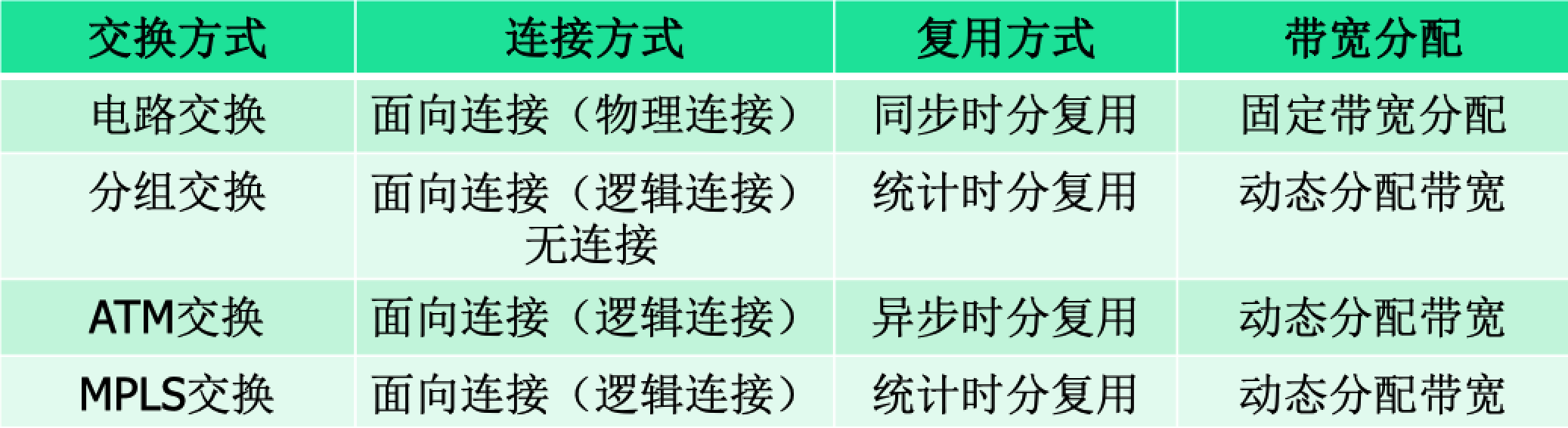## 各种常用网络的交换方式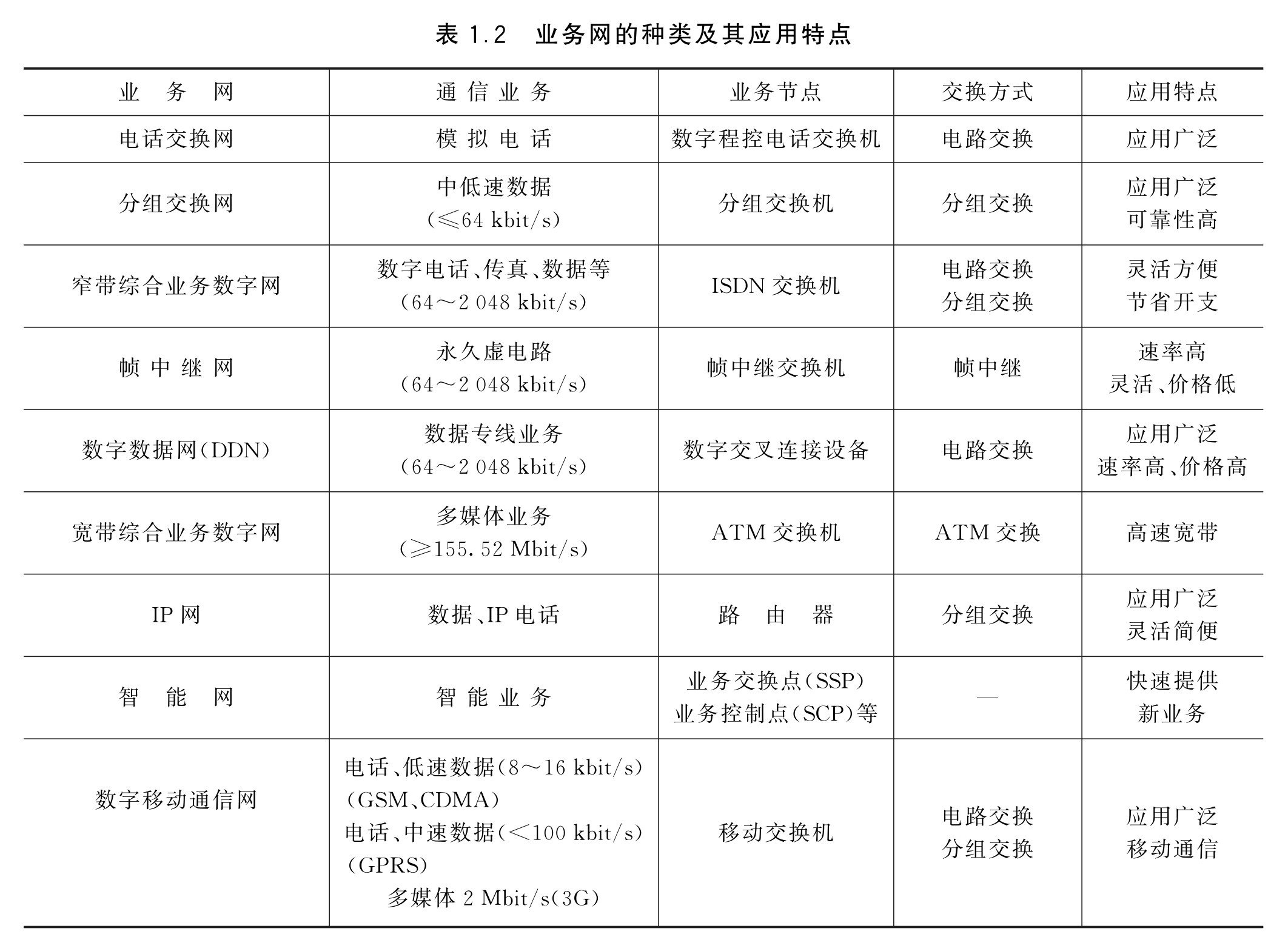GSM 电路
GPRS 电路 & 分组
3G 先电路 & 分组，后纯分组
4G 5G 分组
MPLS 带标记的分组

PSTN No.7
MPLS LDP、SIP
Internet IP
SDN Openflow

## 电信交换系统的基本结构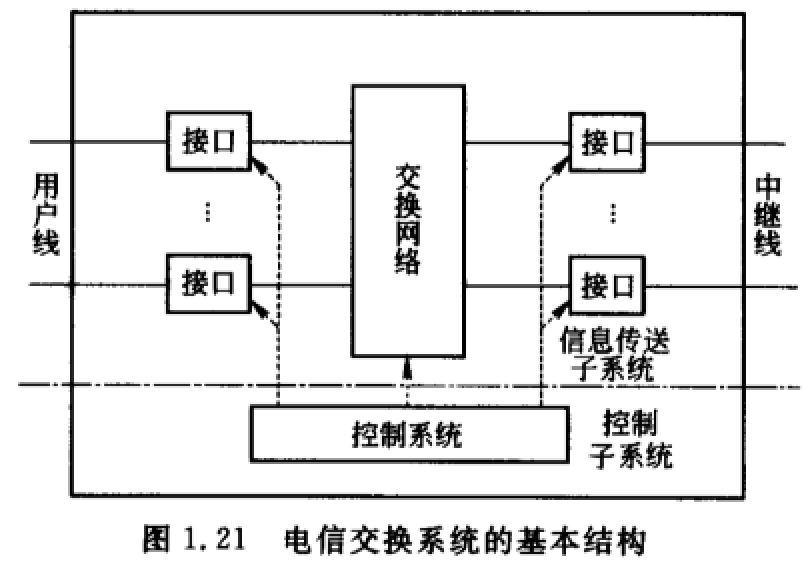• 信息传送子系统：
• 交换网络：完成交换，实现任意出线与入线的互联。
• 各种接口：分为用户接口和中继接口，用途是进行信号转换。
• 控制子系统：由处理机系统构成，是交换系统的“指挥中心”。

# 第二章 交换网络

## 连接概念

### 连接、连接方式

• 集合描述法：所有入线序号为集合$T$，出线序号为集合$R$。连接$c = \{t,R_t\}, t \in T , R_t \subset R$
• 函数描述法：$f(t)=R_t,\space R_t \subset R$
• 排列表达式：$\begin{pmatrix} t_1 && t_2 && \cdots && t_n \\ r_1 && r_2 && \cdots && r_n \\ \end{pmatrix}$，入线排列表达式$(0,1,2...,N-2,N-1)$
• 图形表示法：略
• 二进制函数表示：$E(x_{n-1}x_{n-2}...x_1x_0)=x_{n-1}x_{n-2}...x_1\bar{x_0}$，表示点到点二进制编号。

• 直线连接：略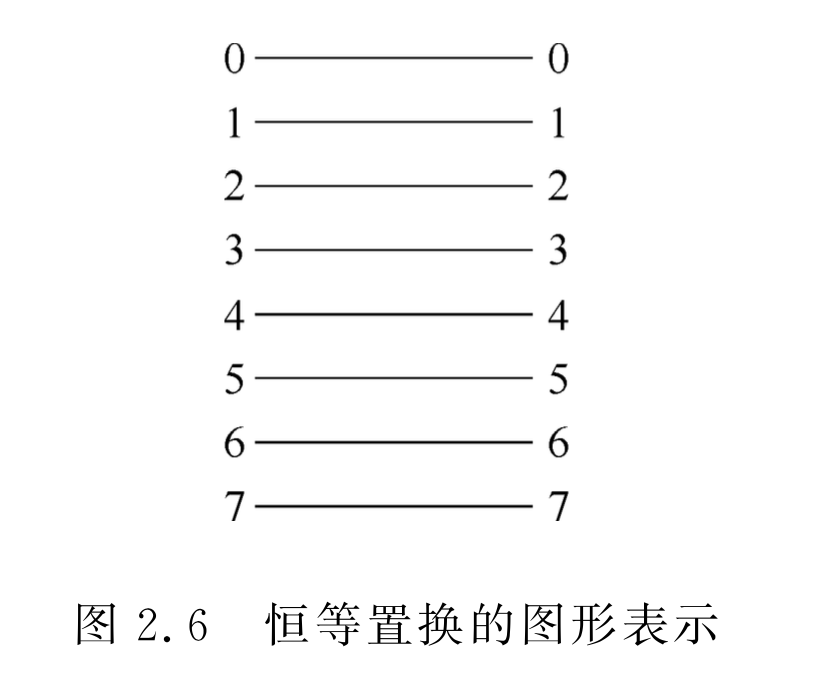• 交叉连接（交换置换）：$(1,0,3,2,...,N-1,N-2)$$E(x_{n-1}x_{n-2}...x_1x_0)=x_{n-1}x_{n-2}...x_1\bar{x_0}$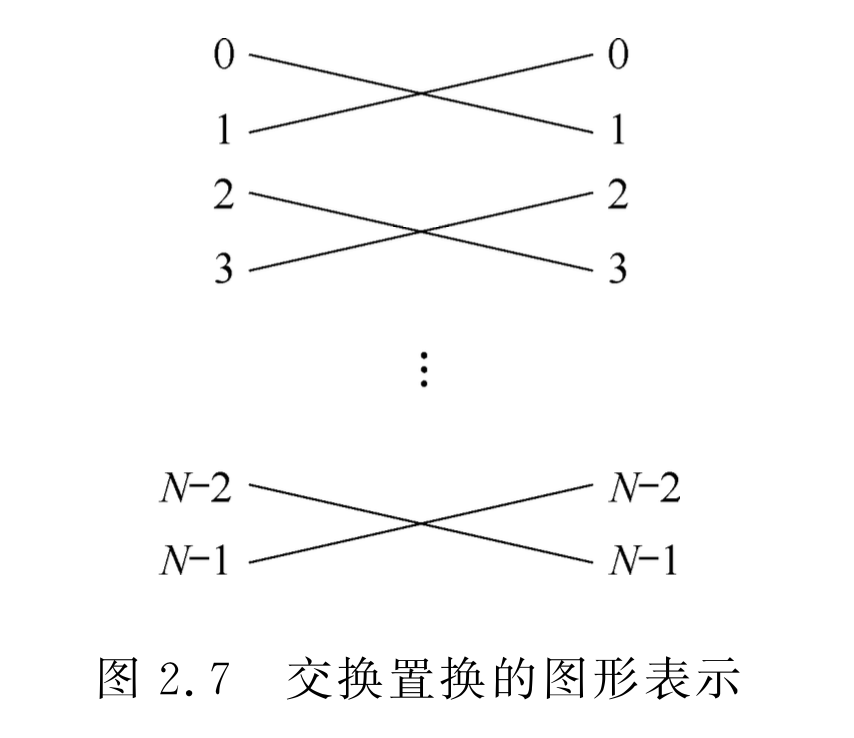• 蝶式连接：$\beta (x_{n-1}x_{n-2}...x_1x_0)=x_0x_{n-2}...x_1x_{n-1}$，将输入端二进制最高位$x_{n-1}$与最低位$x_0$互换位置得到。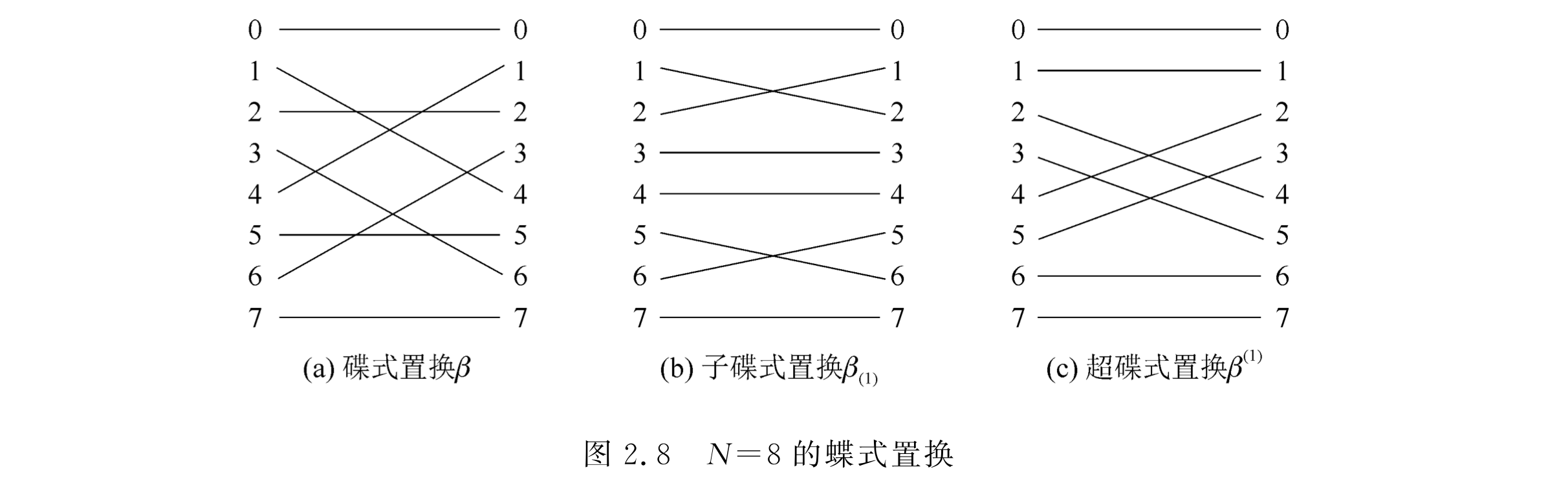• 均匀洗牌置换：将入线均分，前一部分和后一部分按顺序一个接一个交叉连接。$\delta(x_{n-1}x_{n-2}...x_1x_0)=x_{n-2}x_{n-3}...x_1x_0x_{n-1}$，将入线二进制地址循环左移一位得到。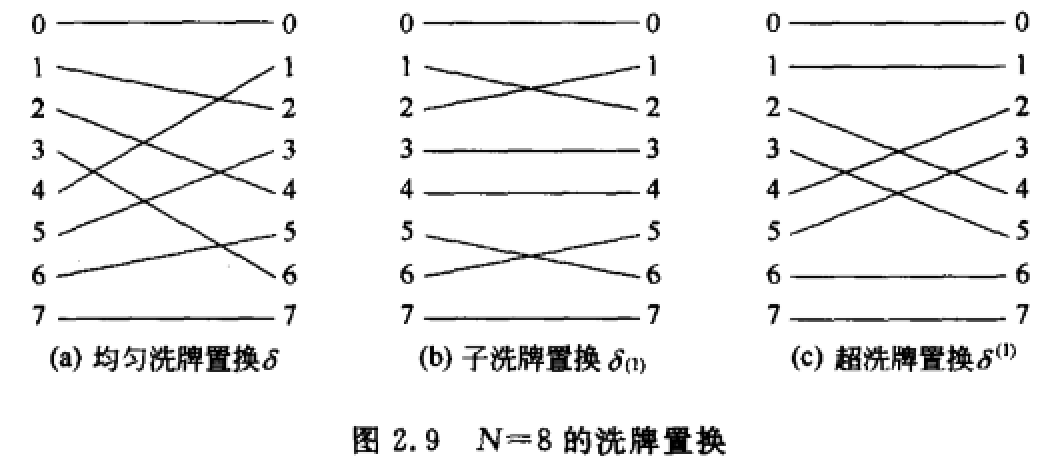• 间隔交叉连接（方体置换）：实现二进制地址编号中第 k 位位置不同的输入端和输出端之间的连接。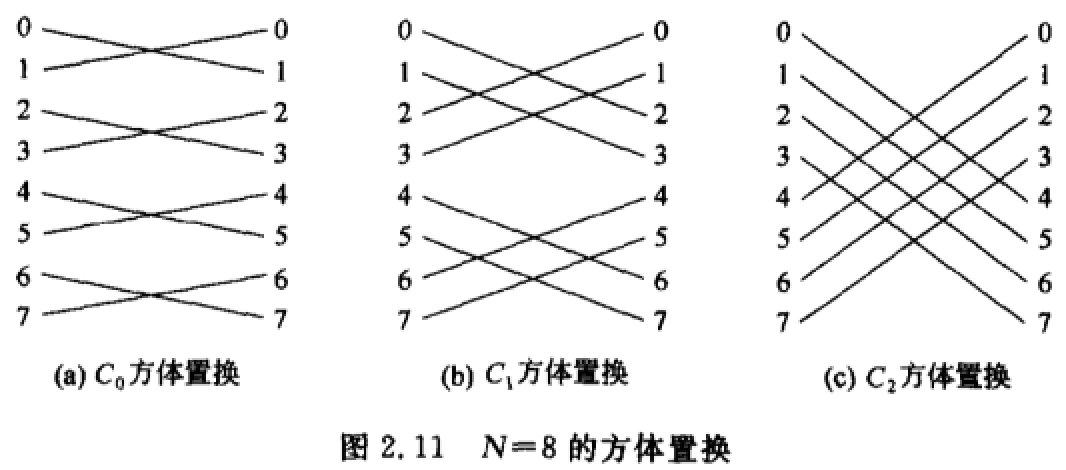### 点对点、同发、广播

• 如果$R_t$只有唯一元素，为点到点连接。

• 如果$R_t$包含多个元素，为点到多点连接。

• 如果$R_t \ne R$，为同发。
• 如果$R_t = R$，为广播。$N!$（1）$N!C_M^N$ （2）$M^N$

## 交换单元的结构、特性、工作原理

### 交换单元的分类

• 入线和出线信息传送方向：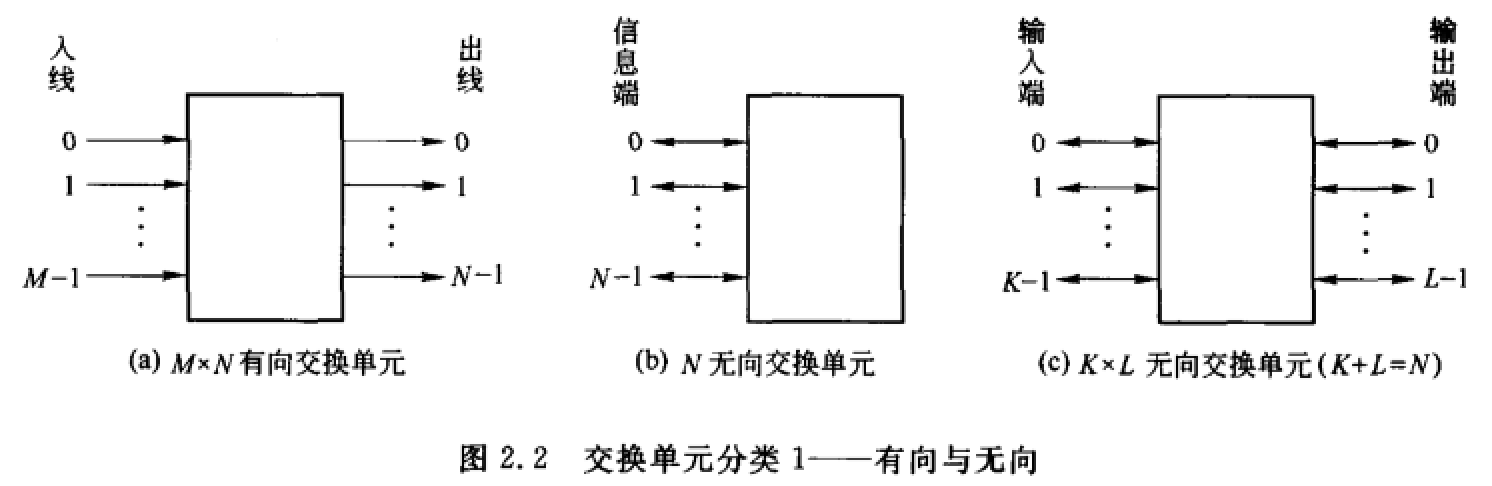• 入线和出线的数量关系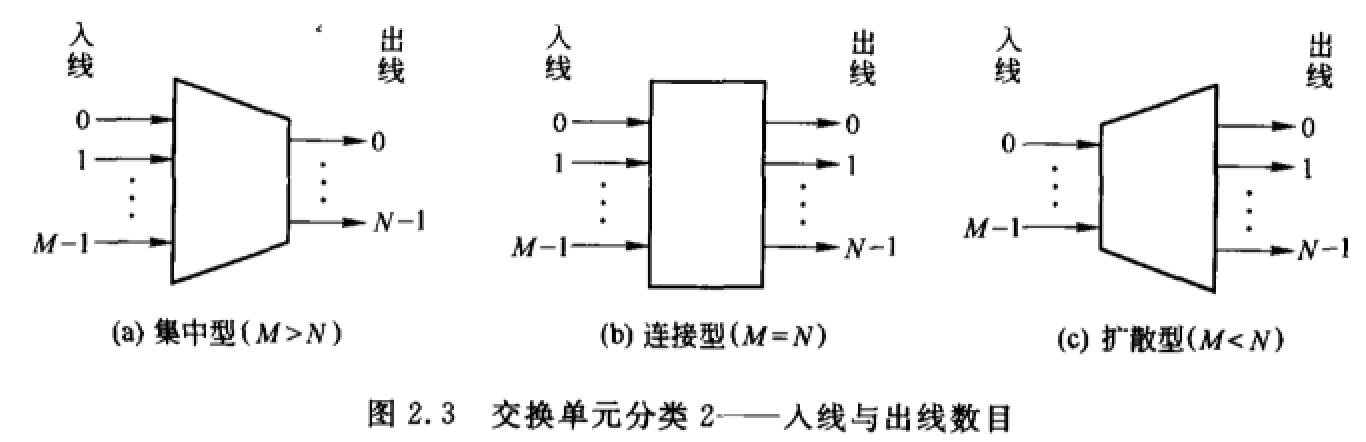• 时分与空分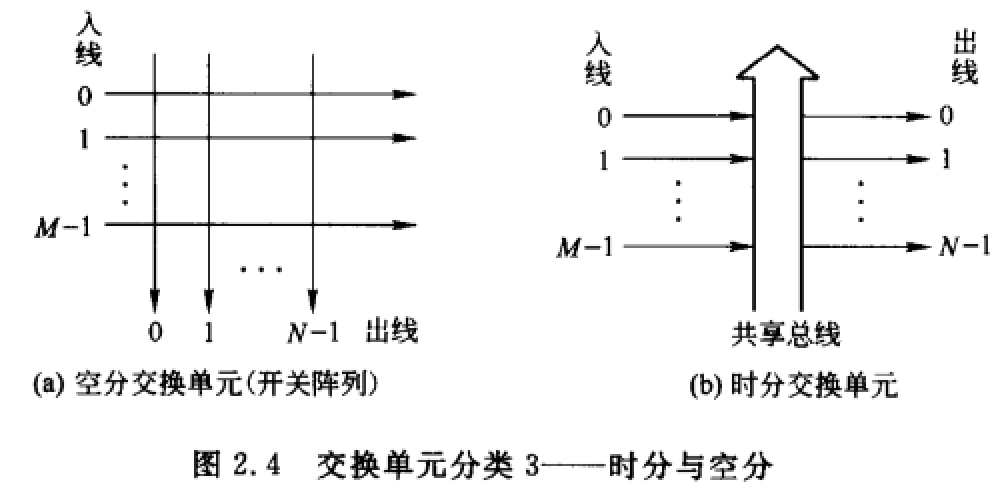• 数字/模拟### 基本交换单元与开关阵列

• $M\times N$ 有向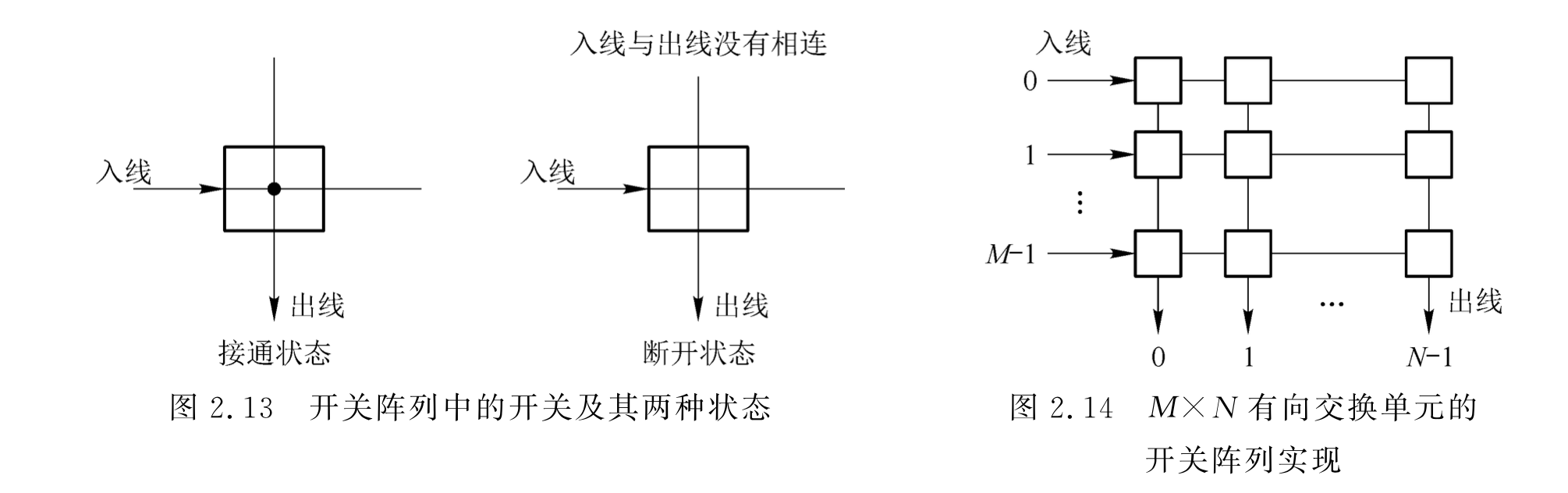采用矩阵表示开关通断：

$\begin{pmatrix} 1 && 0 && \cdots && 0 \\ 0 && 0 && \cdots && 1 \\ \vdots && \vdots && \ddots && \vdots \\ 0 && 1 && \cdots && 0 \end{pmatrix}$

不允许出线冲突时，同 column 只允许一个 1 出现；不允许同发广播时，同 row 只允许一个 1 出现。

• $N$ 无向

特点是主对角线不连通。需要$N(N-1)/2$个双向开关，或者$N(N-1)$个单向开关。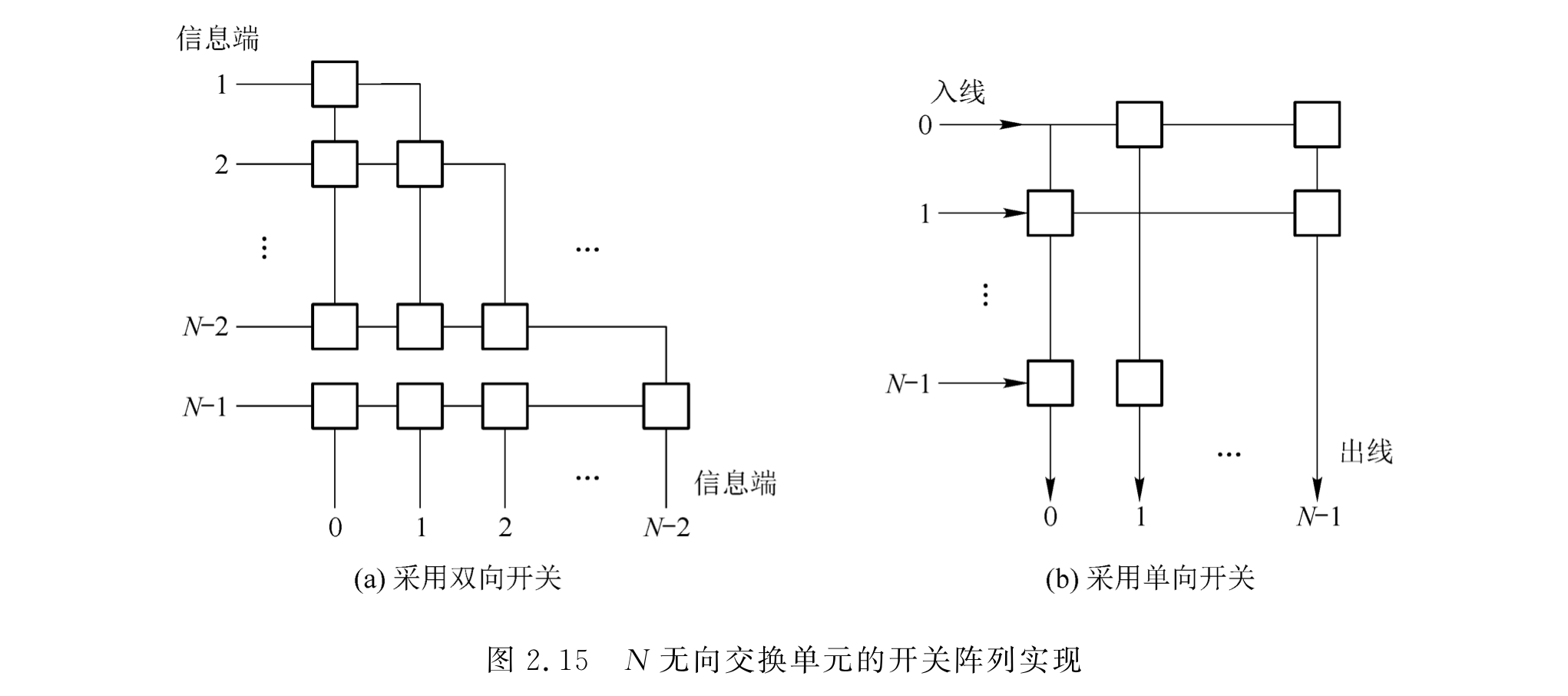• $M\times N$ 无向

需要$M \times N$个双向开关，或者$2 \times M \times N$个单向开关。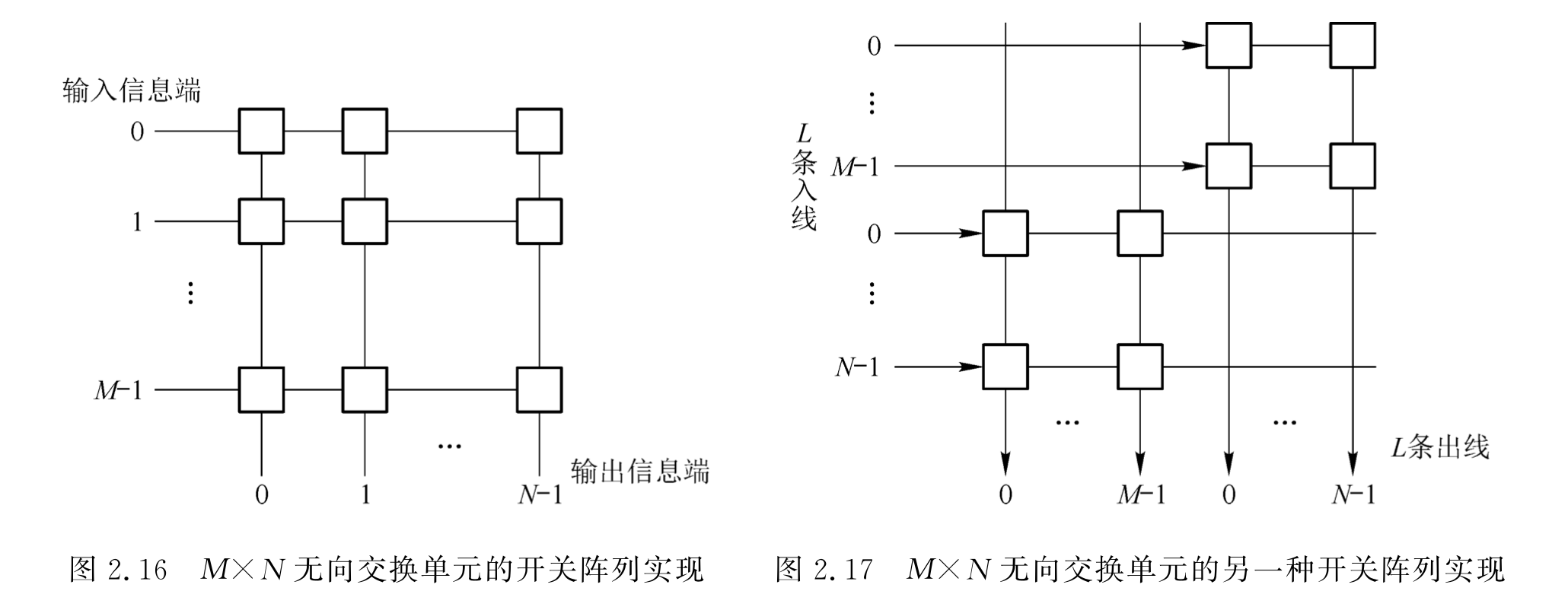#### 开关阵列性质

• 空分交换。
• 容易实现同发和广播。
• 信息从入线到出线具有均匀的单位延迟时间：信息从任一入线到任一出线经过的开关数是相等的，不存在时延抖动。
• 开关阵列的控制简单。
• 适用于构成较小规模的交换单元。
• 开关阵列的性能取决于所用的开关性能。（继电器、模拟电子开关、数字电子开关）

• 可用多路选择器。
• 可用 22 交叉连接单元（crossbar）：可以避免出线冲突，但不支持同发。

### S 接线器

S 和 T 接线器仅用于 PCM（数字）交换。

• 构成：

• 交叉点矩阵（开关阵列）。
• 一组控制存储器（CM，Control Memory）。
• 功能：输入输出线之间的空间交换。

• 规格

• 一个 S 接线器所含控制存储器 CM 的数量=入（出）线数。（输入控制 CM 数=入线数，反之）
• 每个控制存储器 CM 含有的存储单元个数=时隙数。
• 每个存储单元中存储索引存储单元的地址，为$m$位 bit，且满足$N \le 2^m$，其中$N$为接线器的入（出）线数。
• CM 具有输入控制方式与输出控制方式：

• 输入控制：控制一条输入线与每一条输出线的交叉点的开启与闭合，存储单元的内容表示对应输入复用线与哪一个输出复用线的交叉点开关在该存储单元所对应的时隙内接通（对于入线$a$，在当前时隙$TS_i$，从$CM_a$$i$号存储单元读出需要交换到的出线$b$，打开输入线$a$$b$相交叉的开关，完成空间交换）
• 输出控制：控制一条输出线与每一条输入线的交叉点的开启与闭合，存储单元的内容表示对应输出复用线上哪个交叉点在该存储单元所对应的时隙内接通（对于出线$b$，在当前时隙$TS_i$，从$CM_a$$i$号存储单元读出接受输入的入线$a$，打开输入线$a$$b$相交叉的开关，完成空间交换）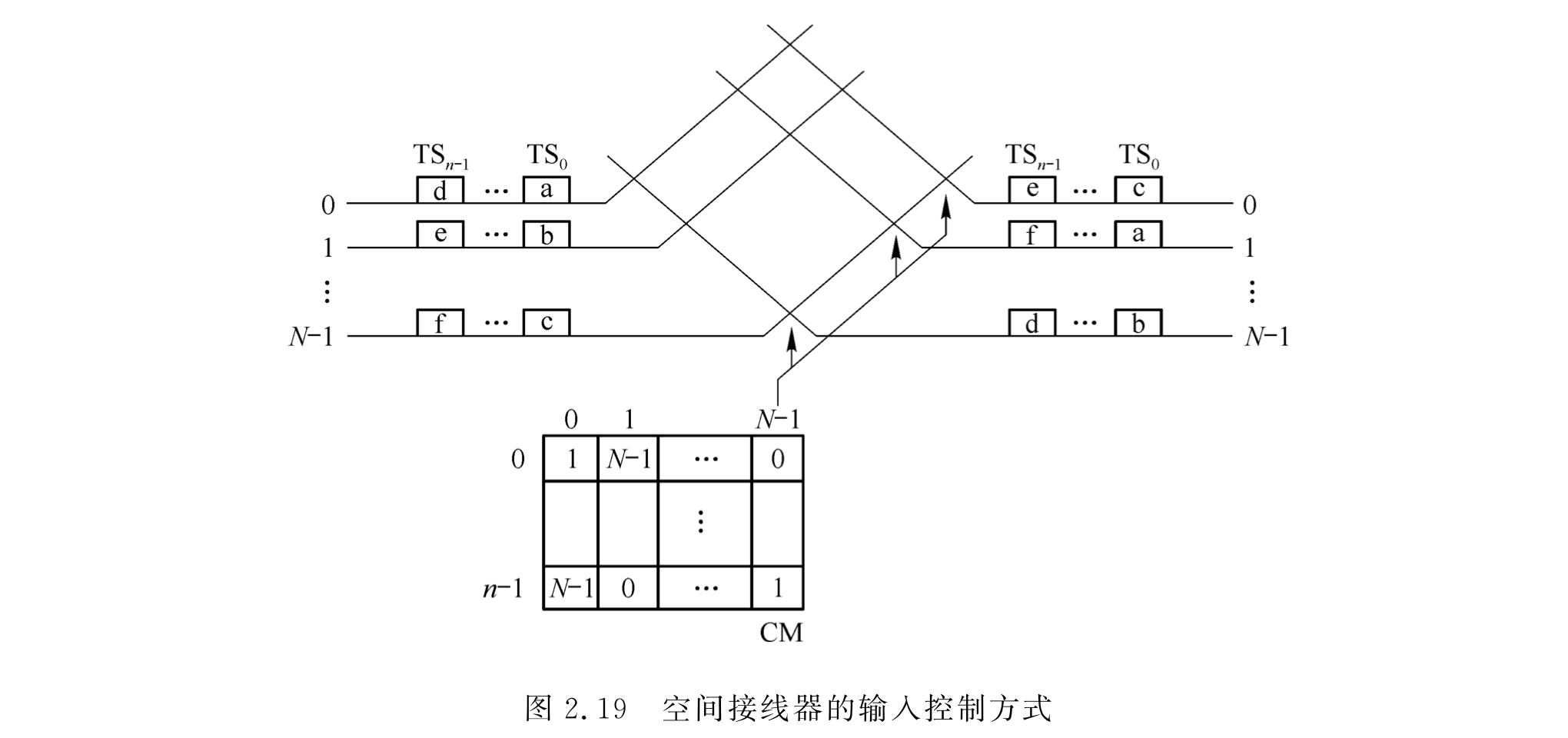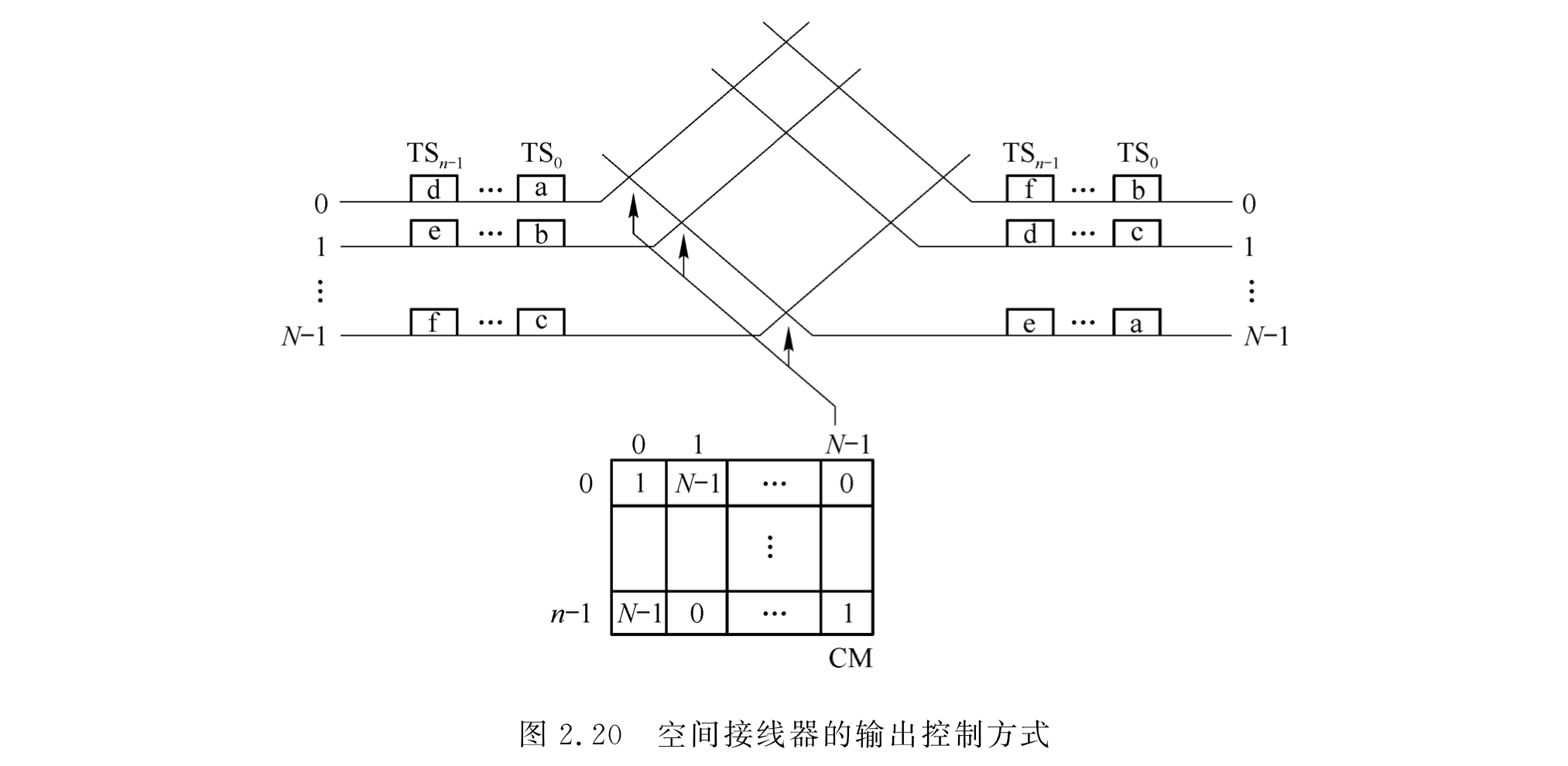• 只完成空间交换，不进行时隙交换。
• 空间接线器按时分方式工作。
• 控制方式易于实现多播（将多个 CM 的$TS_i$的存储单元置为同一条输入线即可）。
• 输出控制方式可自动避免出线冲突。### T 接线器

• 共享存储器型：共$N$个出入线缓存。入线缓存控制读出、出线缓存控制写入。

• 共享总线型：总线按照时隙轮流分配给入线控制部件和出线控制部件使用。

• $kNV=B/T$$N$为入线数、$V$为入线速率、$B$为每时隙传送比特数、$T$为时隙长度，$k$为时隙分配因子）

• 话音存储器（SM，Speech Memory）：暂存话音的数字编码信息。
• 存储单元个数等于 PCM 线路每帧时隙数；每单元至少 8bit（一路话音）。
• 控制存储器（CM，Control Memory）：
• 存储单元个数等于 SM；每单元至少$log_2n（n 为时隙数）$比特。

• SM：前半周期顺序写入话音、后半周期从地址读出话音

• CM：前半周期顺序写入控制地址、后半周期控制读出话音。

缺点：复用级别越高，对控制电路要求越高，无法达成很大规模；时隙转换延迟不定。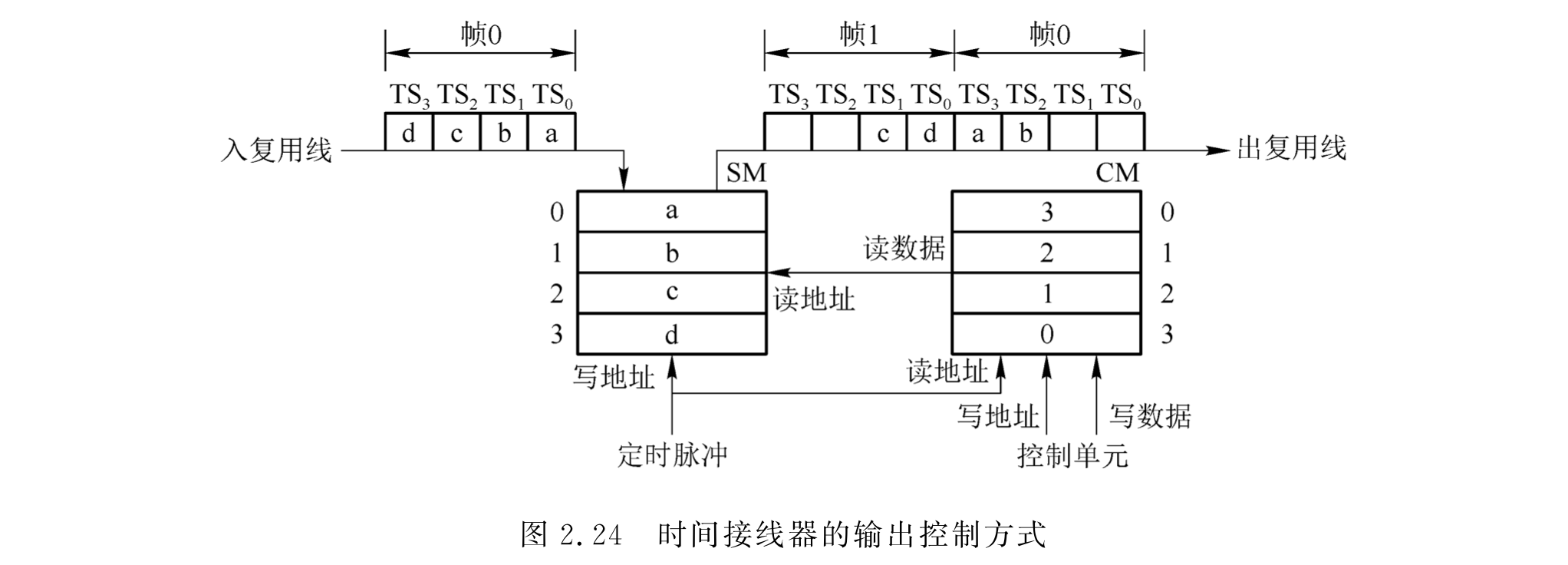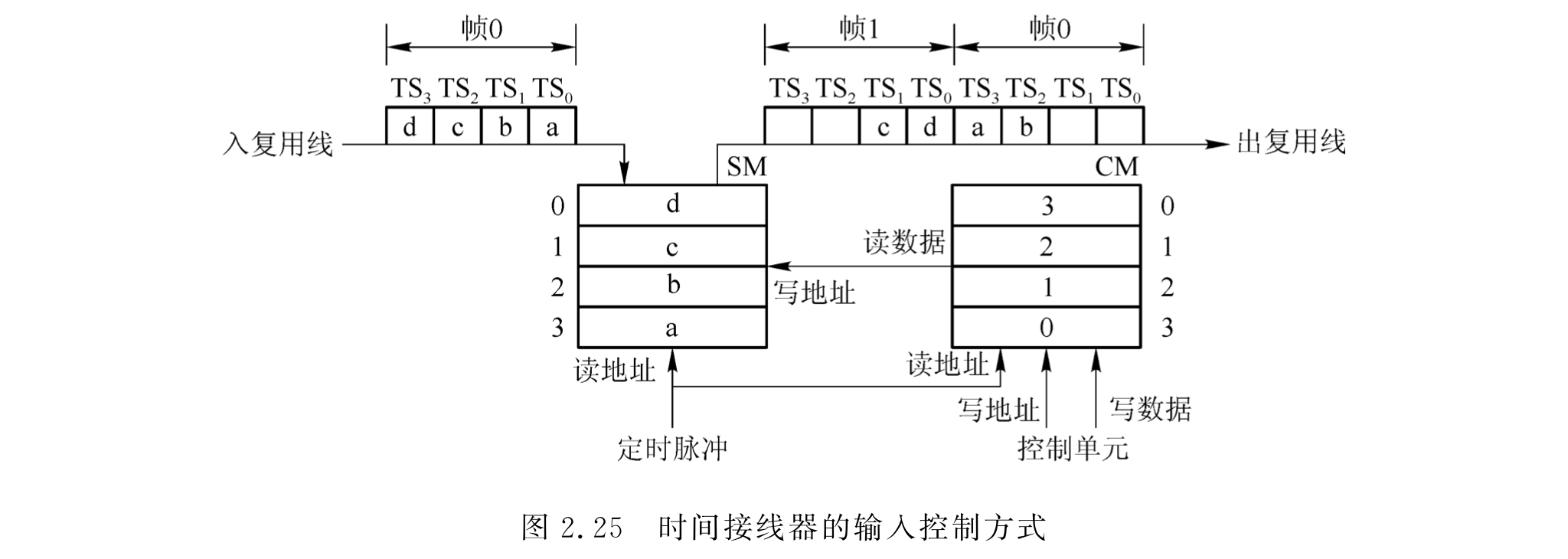• 时分交换，用于交换同步时分复用的信号。
• SM 划分为$N$个区域，每个区域一个字节，存放一个话音数据。各个区域间不共享，无排队缓冲。
• 交换的控制过程由 CM 硬件实现，速度快。
• 交换时延小且稳定。
• 严格无阻塞，并且可避免出线冲突。
• 易于实现同发和广播。
• 速率固定。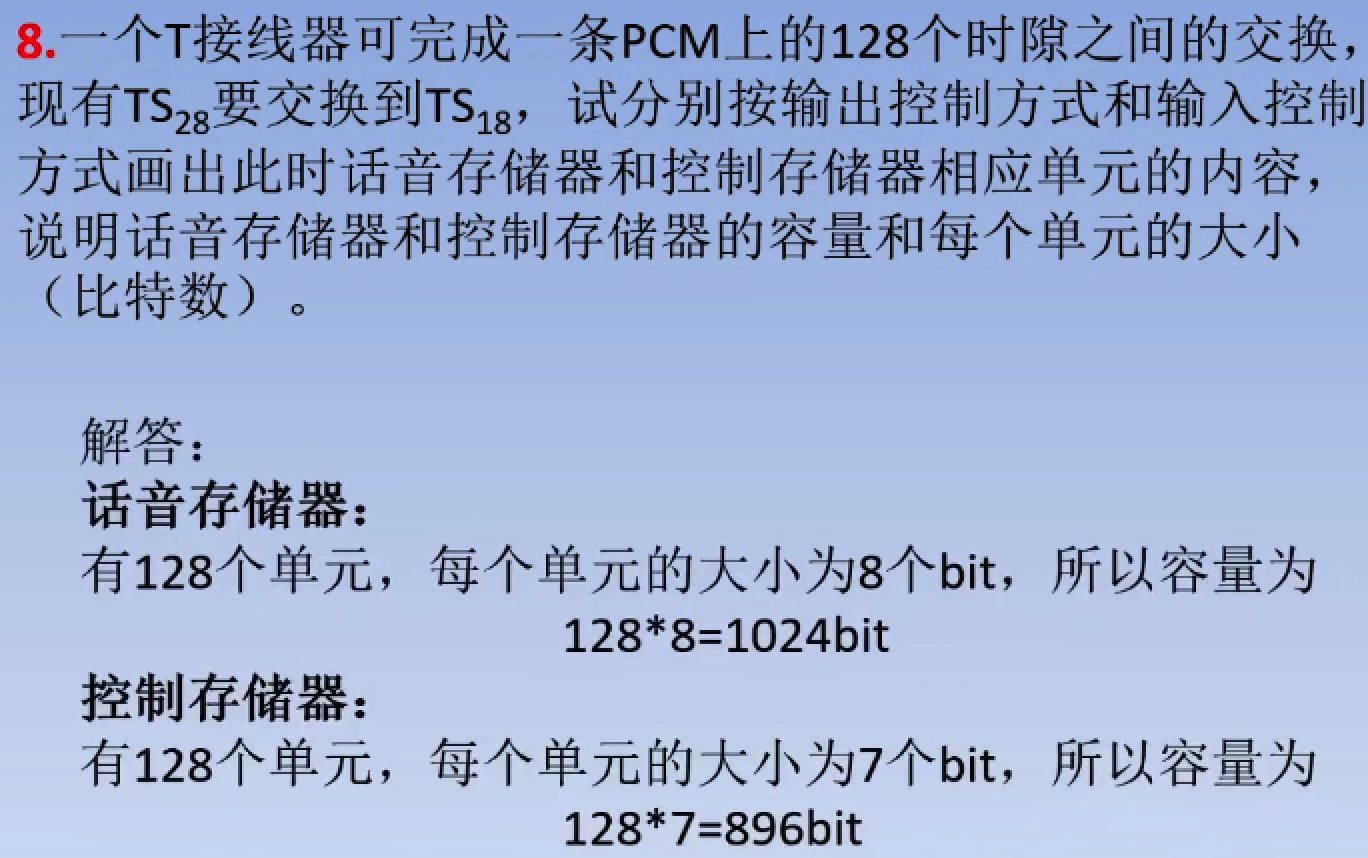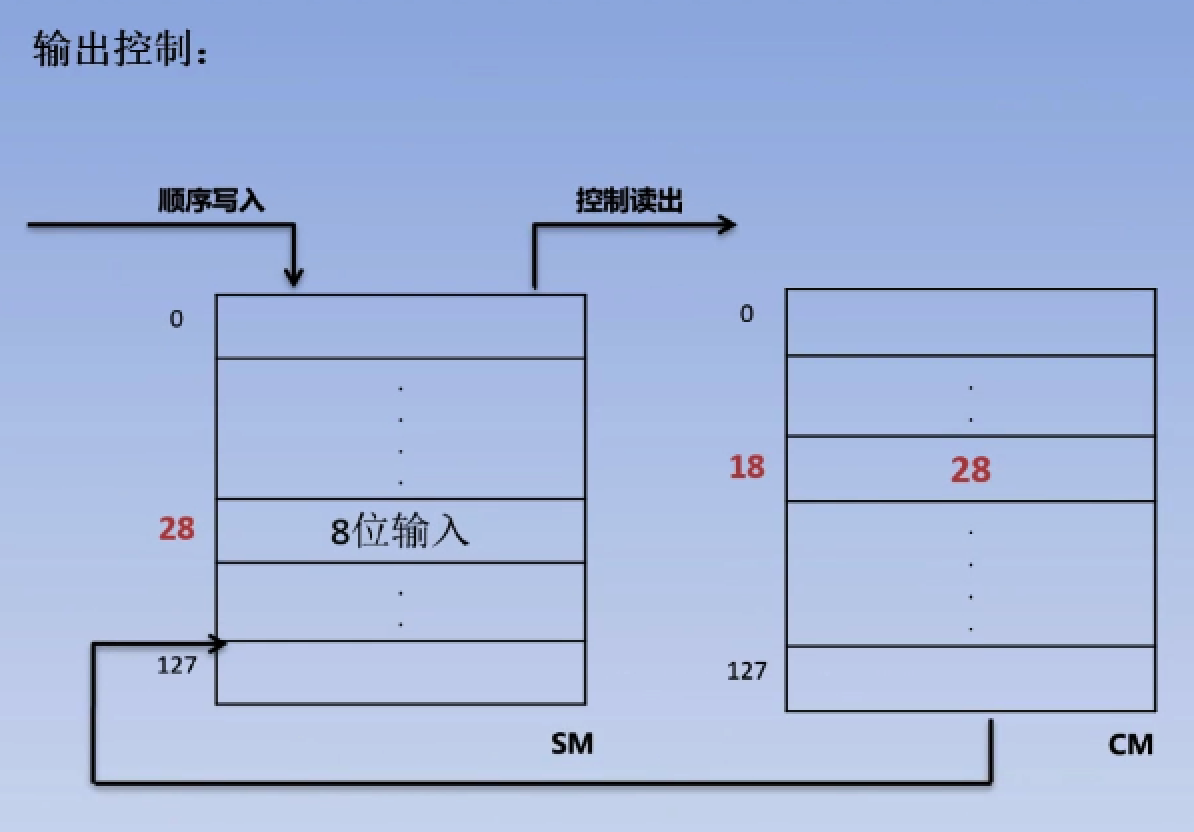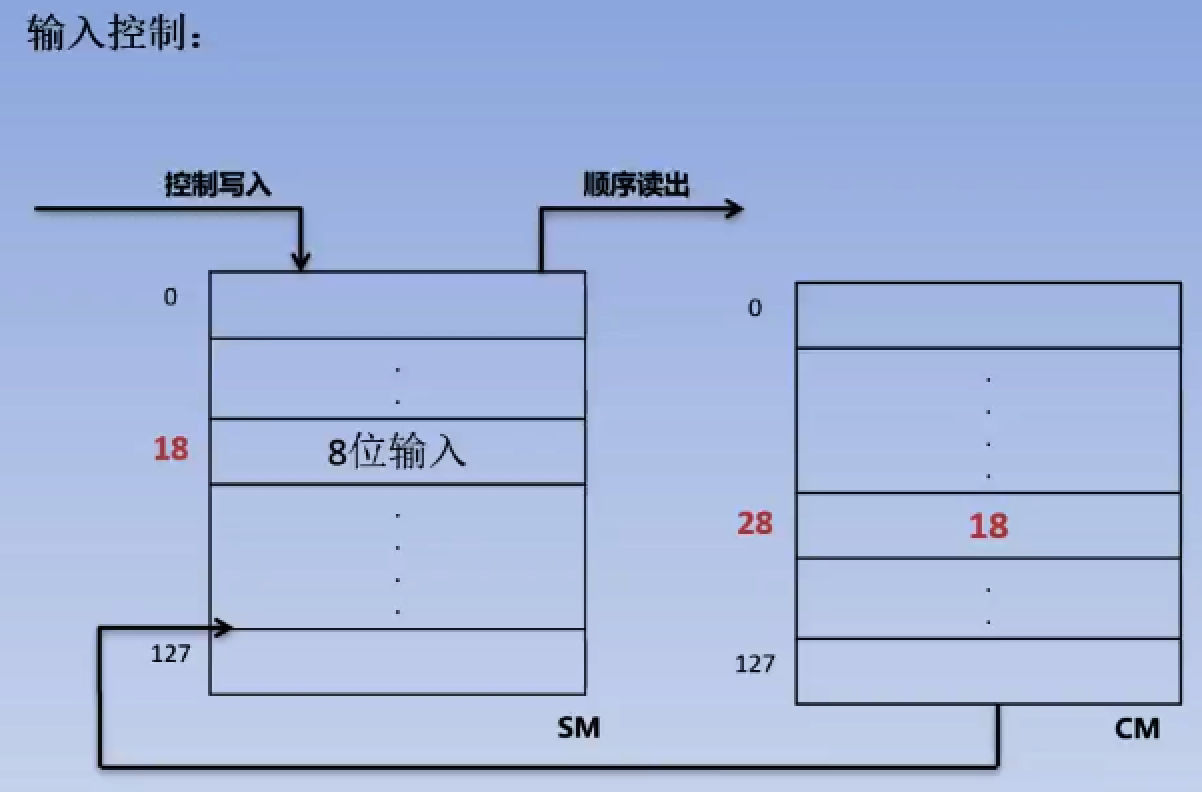## 交换网络的分类与特性

• 单级网络、多级网络：需要交换的信息从交换网络入线到出线经过 n 个交换单元，并且同一级中不同交换单元的入线和出线之间可以建立连接（交换单元需要发挥交换作用而非直连），则称为 n 级交换网络。
• 有阻塞网络、无阻塞网络：见上一小节。
• 单通道网络、多通道网络：任一条入线和出线之间只存在唯一的一条通路，则为单通道网络。
• 出线竞争：不同入端来的信息同时争抢交换网络同一个输出端口而发生的竞争。
• 内部竞争：同时要交换的两路信息同抢交换单元内部的通路资源。

### CLOS 网络：严格无阻、可重排无阻公式和构造

3 级 CLOS 严格无阻$m \ge 2n-1$

3 级 CLOS 可重排无阻$m \ge n$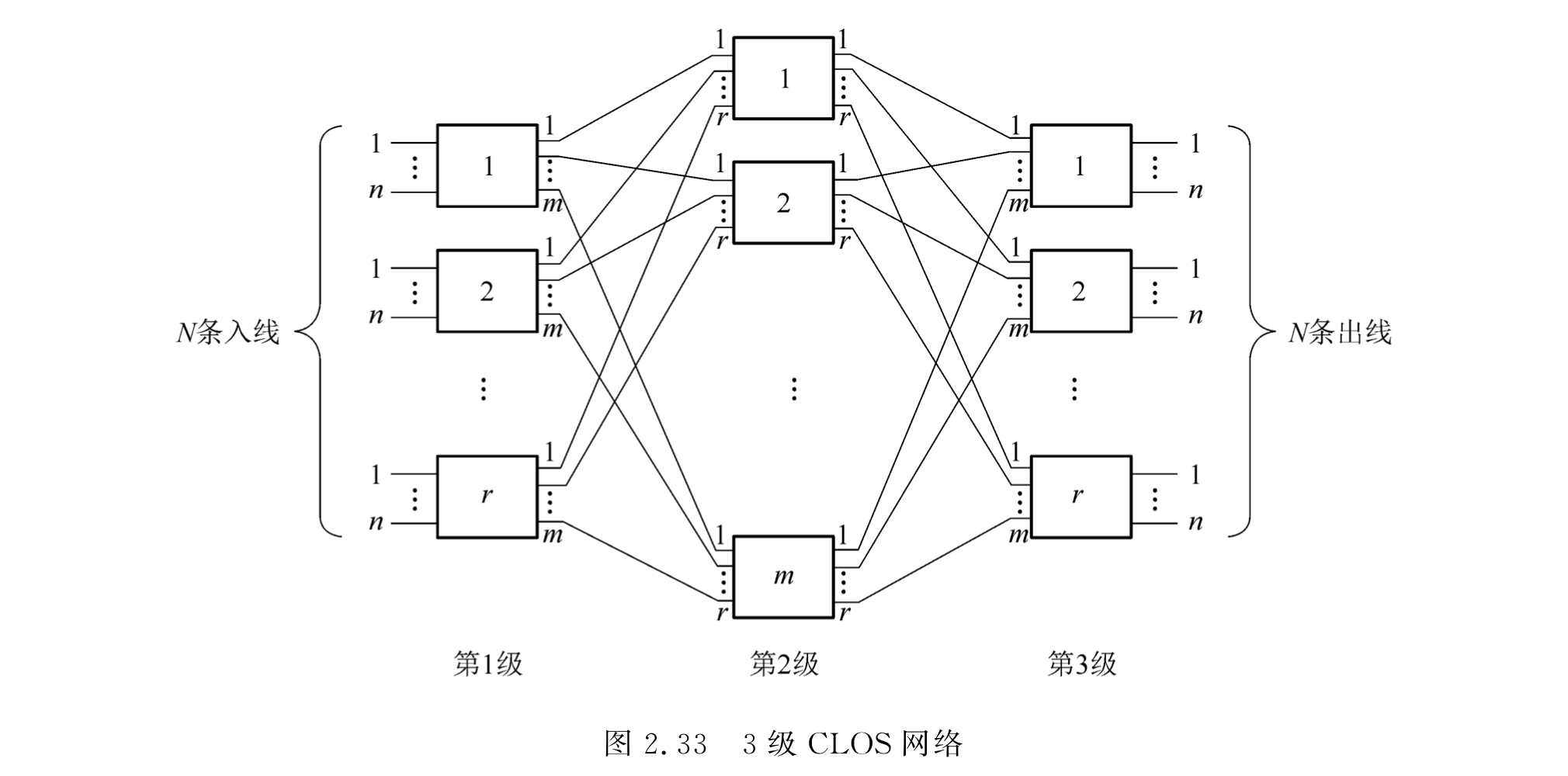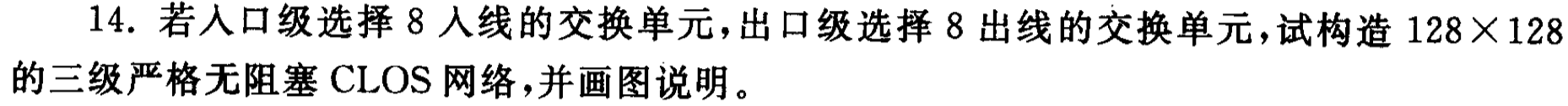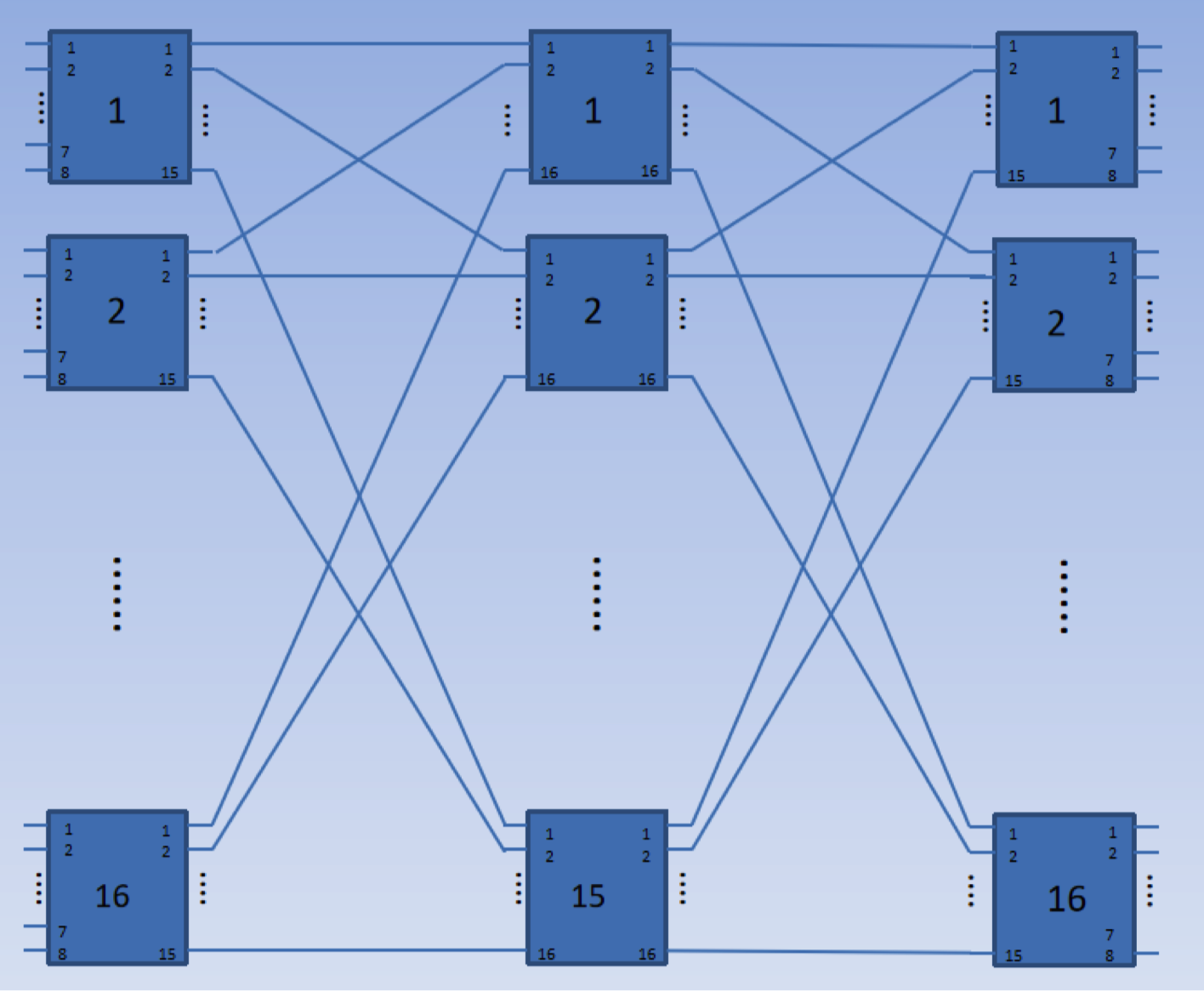### TST：结构、SM 和 CM 容量和内容、反向半帧、同发特性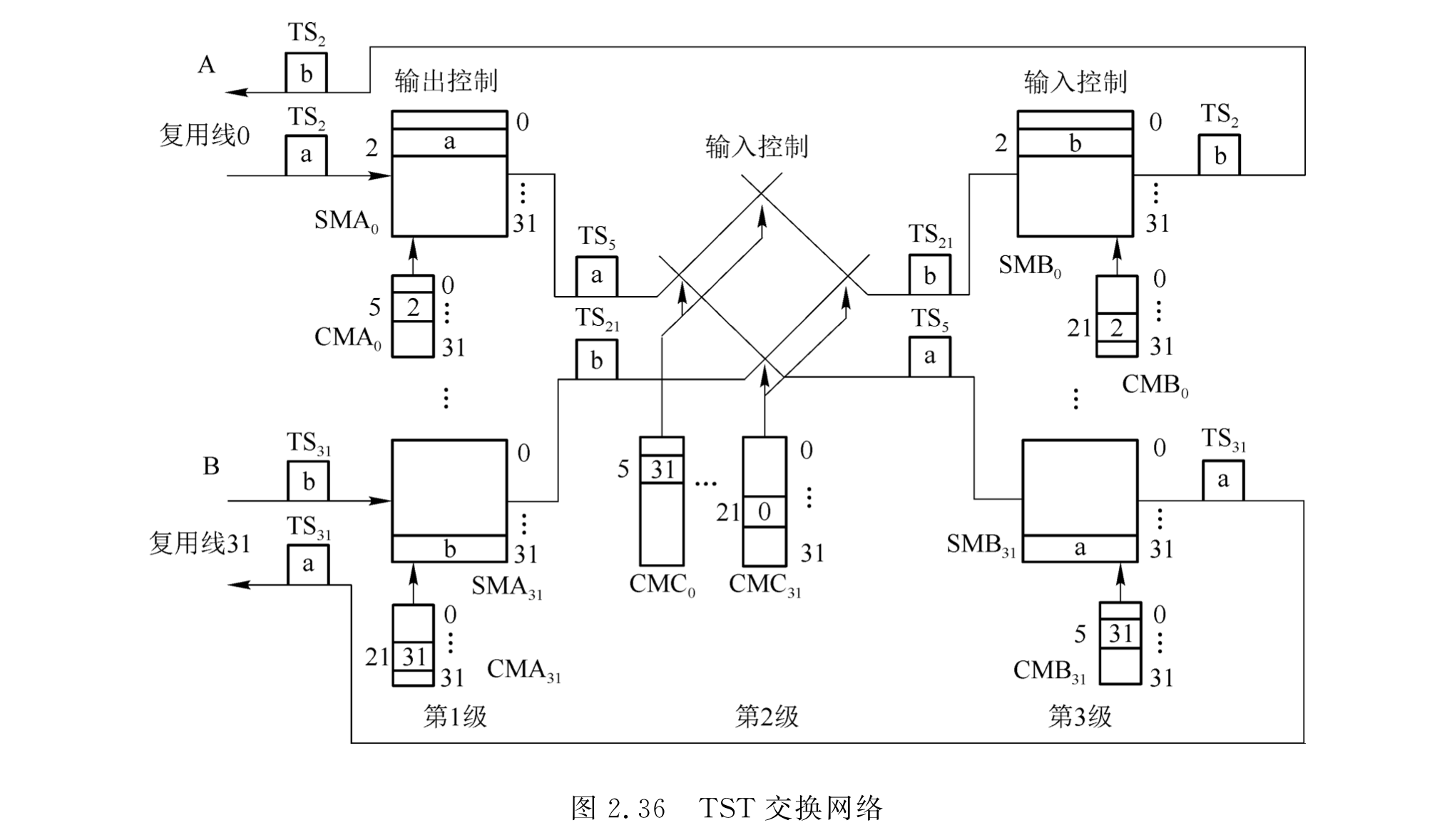TST网络完全无阻塞的条件：m（内部时隙数）=2n（输入时隙数）

• 初 T 顺入控出， S 输出控制/输入控制， 次 T 控入顺出。
• 初 T 控入顺出， S 输出控制/输入控制， 次 T 顺入控出。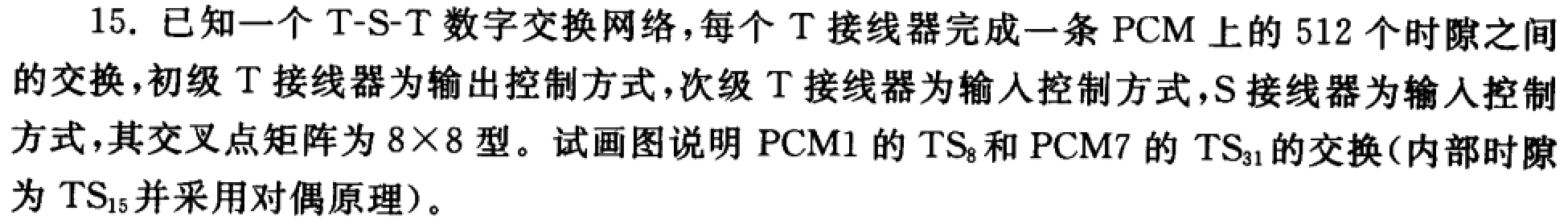### BANYAN 网络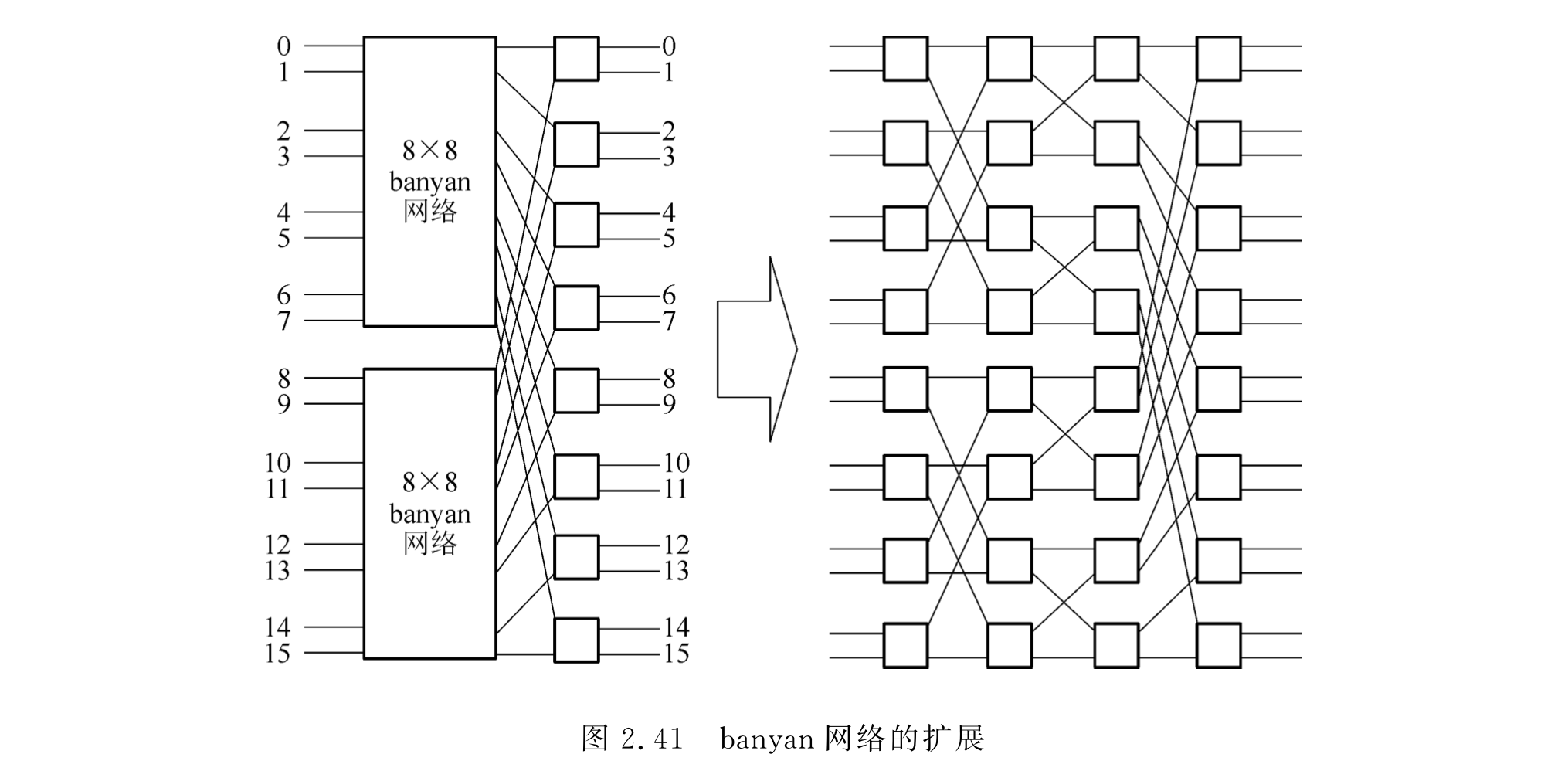• 增加网络级数（如 BENES 网络），每增加一级，通路数翻倍。但同时失去唯一路径和自选路特性。
• 使用扩展型或膨胀型 BANYAN 网络。
• BATCHER-BANYAN（排序-BANYAN）网络：严格无阻塞、结构规整、自动选路。
• 减少入线的信息量，加大入线缓存。
• 增加平面数，构造多通道交换网络。（几平面就是几倍的交换单元）

### BENES 网络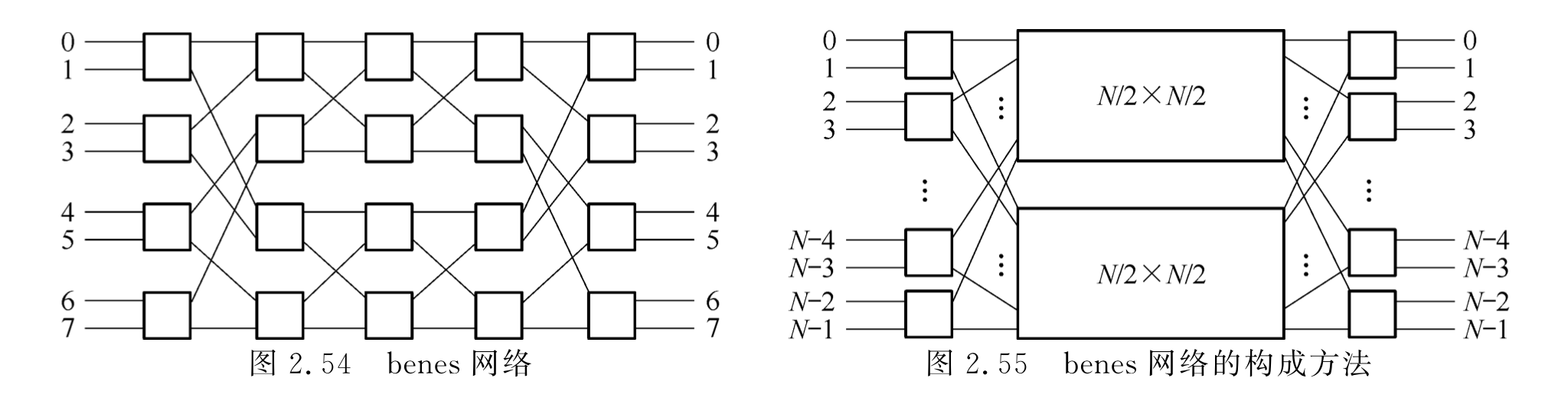$2\times2$交换单元构成$16\times16$的 BANYAN 网络和 BENES 网络，比较二者异同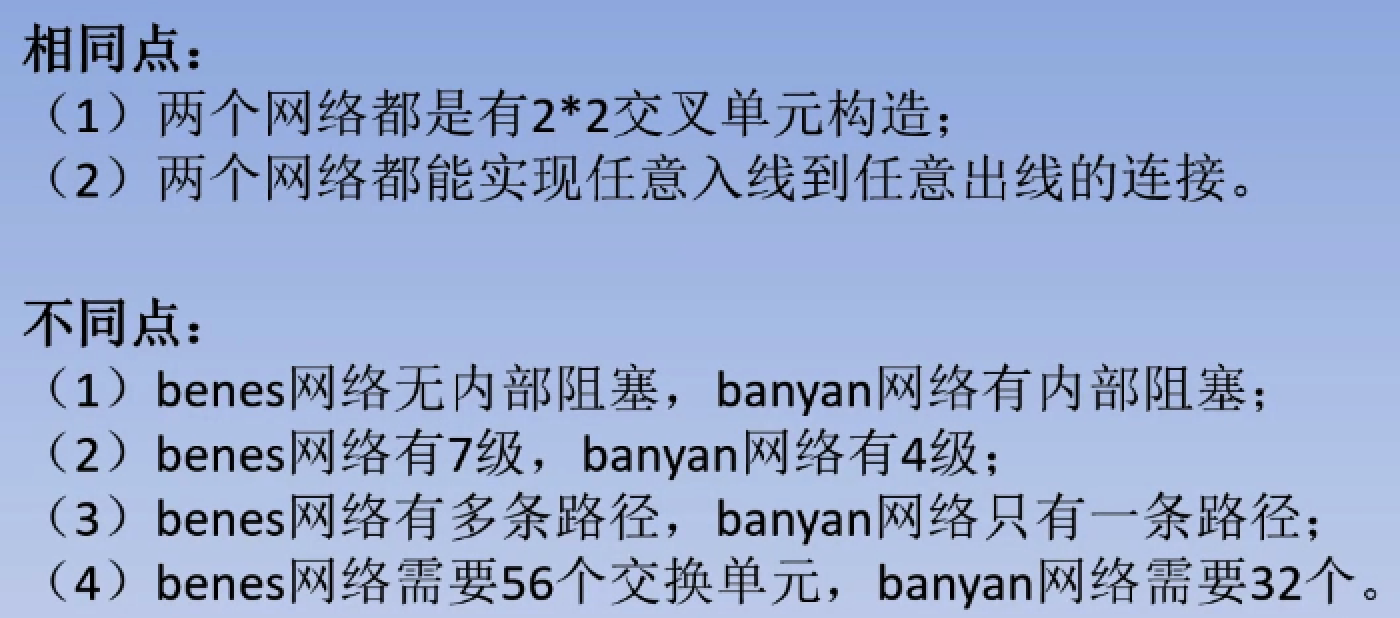xqmmcqs

2022-06-12

2022-07-11